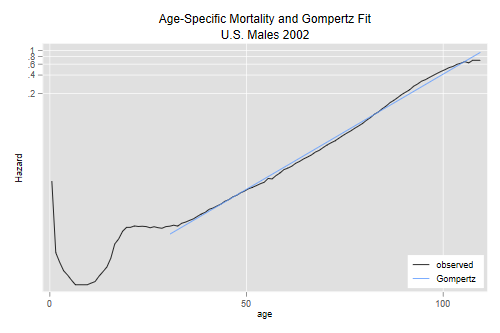Germán Rodríguez
Demographic Methods Princeton University## The Gompertz Model

We will illustrate fitting a Gompertz model to U.S. adult mortality. The survival function for U.S. males in 2002 is available in the datasets section of this website.

```. infile age lx using ///
>   https://grodri.github.io/datasets/us2002m.dat, clear
```
```> us <- read.table("https://grodri.github.io/datasets/us2002m.dat",
+   header = FALSE, col.names = c("age", "lx"))
```

We will compute the cumulative hazard by taking logs, the average hazard for each age by differencing, and the age midpoint by averaging:

```. quietly replace lx = lx / 100000

. gen Hx = - log(lx)

. gen hx = H[_n+1] - H
(1 missing value generated)

. gen am = (age + age[_n+1])/2
(1 missing value generated)

. line hx am, yscale(log)
``````> library(dplyr)
> library(ggplot2)
> us <- mutate(us, lx = lx/100000, Hx = -log(lx))
> midpoints <- function(x) (x[-1] + x[-length(x)])/2
> ush <- data.frame(hx = diff(us\$Hx), x = midpoints(us\$age))
> ggplot(ush, aes(x, hx)) + geom_line() + scale_y_log10()
```We see the familiar shape and note that it is quite linear for adults, as shown by the red line based on a Gompertz fit. How do we get that?

We just regress the log-hazard on age for ages 30 and older. To make the constant a bit more meaningful we will measure age from 30

```. gen loghx = log(hx)
(1 missing value generated)

. gen am30 = am - 30
(1 missing value generated)

. regress loghx am30 if age >= 30

Source │       SS           df       MS      Number of obs   =        80
─────────────┼──────────────────────────────────   F(1, 78)        =  21039.26
Model │  314.127049         1  314.127049   Prob > F        =    0.0000
Residual │  1.16458015        78  .014930515   R-squared       =    0.9963
Total │  315.291629        79  3.99103328   Root MSE        =    .12219

─────────────┬────────────────────────────────────────────────────────────────
loghx │ Coefficient  Std. err.      t    P>|t|     [95% conf. interval]
─────────────┼────────────────────────────────────────────────────────────────
am30 │   .0858109   .0005916   145.05   0.000     .0846331    .0869886
_cons │  -6.889114   .0273242  -252.12   0.000    -6.943512   -6.834716
─────────────┴────────────────────────────────────────────────────────────────
```
```> lf <- lm(log(hx) ~ I(x - 30), data = filter(ush, x > 30))
> summary(lf)

Call:
lm(formula = log(hx) ~ I(x - 30), data = filter(ush, x > 30))

Residuals:
Min       1Q   Median       3Q      Max
-0.29936 -0.10847 -0.00201  0.09795  0.29813

Coefficients:
Estimate Std. Error t value Pr(>|t|)
(Intercept) -6.8891145  0.0273241  -252.1   <2e-16 ***
I(x - 30)    0.0858109  0.0005916   145.1   <2e-16 ***
---
Signif. codes:  0 '***' 0.001 '**' 0.01 '*' 0.05 '.' 0.1 ' ' 1

Residual standard error: 0.1222 on 78 degrees of freedom
Multiple R-squared:  0.9963,	Adjusted R-squared:  0.9963
F-statistic: 2.104e+04 on 1 and 78 DF,  p-value: < 2.2e-16
```

We get an R-squared of 0.9963 and estimates of -6.889 for the constant and 0.0858 for the slope.

The constant tells us the hazard at exact age 30 is just about one per thousand, and the slope tells us that the hazard increases 8.58% per year starting at age 30 (so it doubles in just over 8 years.)

The code used to add the red line to the plot was

```. predict loghf
(option xb assumed; fitted values)
(1 missing value generated)

. gen hf = exp(loghf)
(1 missing value generated)

. twoway (line hx am) (line hf am if age >=30) ///
> , yscale(log) ytitle(Hazard) xtitle(age) ///
>   title("Age-Specific Mortality and Gompertz Fit") ///
>   subtitle("U.S. Males 2002") ///
>   legend(order(1 "observed" 2 "Gompertz") ring(0) pos(5) col(1))

. graph export usm02g.png, width(500) replace
file usm02g.png saved as PNG format
```
```> ushf <- filter(ush, x > 30) %>%  mutate(hf = exp(fitted(lf)))
> ggplot(ush, aes(x, hx)) + geom_line() + scale_y_log10() +
+   geom_line(data=ushf, aes(x, hf), color = "red")
> ggsave("usm02gr.png", width = 500/72, height = 400/72, dpi = 72)
```

We predict the log-hazard but exponentiate to get a fitted hazard. (We usually get more meaningful labels if we let the software use a log scale.)

The datasets section has the survival function for U.S. females (the file is called `us2002f.dat`). Verify that age-specific mortality at age 30 is much lower for women than men (about half!) and that women seem to age a bit faster than men. But are adult female mortality rates ever higher than men’s?

Note: Updated february 2016 using latest data from the Human Mortality Database.

